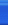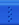# 进阶之路118 Posts :: 3 Stories :: 10 Comments :: 0 Trackbacks

Implement atoi to convert a string to an integer.

Hint: Carefully consider all possible input cases. If you want a challenge, please do not see below and ask yourself what are the possible input cases.

Notes: It is intended for this problem to be specified vaguely (ie, no given input specs). You are responsible to gather all the input requirements up front.

spoilers alert... click to show requirements for atoi.

Requirements for atoi:

The function first discards as many whitespace characters as necessary until the first non-whitespace character is found. Then, starting from this character, takes an optional initial plus or minus sign followed by as many numerical digits as possible, and interprets them as a numerical value.

The string can contain additional characters after those that form the integral number, which are ignored and have no effect on the behavior of this function.

If the first sequence of non-whitespace characters in str is not a valid integral number, or if no such sequence exists because either str is empty or it contains only whitespace characters, no conversion is performed.

If no valid conversion could be performed, a zero value is returned. If the correct value is out of the range of representable values, INT_MAX (2147483647) or INT_MIN (-2147483648) is returned.

//#define INT_MAX 2147483647
//#define INT_MIN -2147483648

double noHeadBlank(const char *str){
double val = 0;
if(str == '-' || str == '+'){
int i = 1;
while(str[i] == '0') ++i;
if(str[i] == '\0') return 0;
else if(str[i] > '0' && str[i] <= '9') {val = str[i] - '0'; ++i;}
else return 0;
for(; str[i] != '\0' && str[i] >= '0' && str[i] <= '9'; ++i)
val = val * 10 + (str[i] - '0');
if(str == '-') val *= (-1);
}else if(str > '0' && str <= '9') {
val = str - '0';
int i = 1;
for(; str[i] != '\0' && str[i] >= '0' && str[i] <= '9'; ++i){
val = val * 10 + (str[i] - '0');
}
}else if(str == '0'){
int i = 1;
while(str[i] == '0') ++i;
if(str[i] == '\0') return 0;
else if(str[i] > '0' && str[i] <= '9') {val = str[i] - '0'; ++i;}
else return 0;
for(; str[i] != '\0' && str[i] >= '0' && str[i] <= '9'; ++i)
val = val * 10 + (str[i] - '0');
}
return val;
}

class Solution {
public:
int atoi(const char *str) {
double val = 0;
if(str == NULL) return 0;
if(str == ' '){
int i = 1;
while(str[i] == ' ') ++i;
if(str[i] == '\0') return 0;
else val = noHeadBlank(str+i);
}else val = noHeadBlank(str);
if(val > (double)INT_MAX) return INT_MAX;
else if (val < (double)INT_MIN) return INT_MIN;
else return (int)val;
}
};


posted on 2014-05-04 19:55 进阶之路 阅读(...) 评论(...) 编辑 收藏